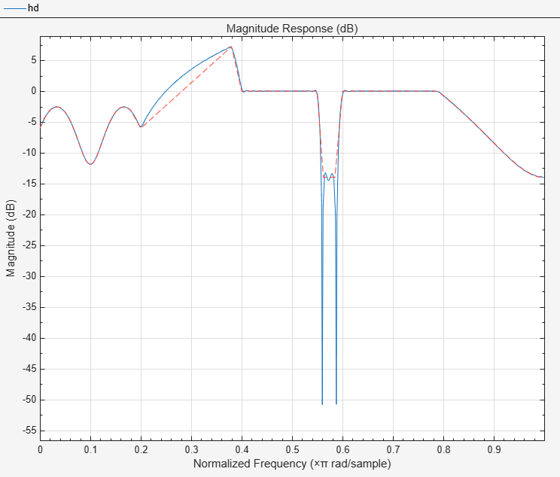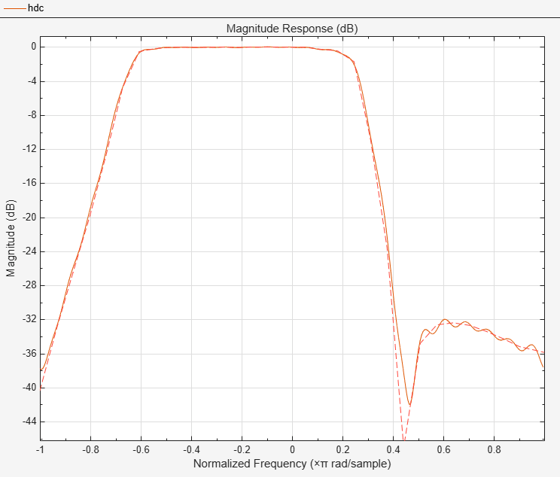Main Content

# freqsamp

Real or complex frequency-sampled FIR filter from specification object

## Syntax

```hd = design(d,'freqsamp','SystemObject',true) hd = design(...,'filterstructure',structure,'SystemObject',true) hd = design(...,'window',window,'SystemObject',true) ```

## Description

`hd = design(d,'freqsamp','SystemObject',true)` designs a frequency-sampled filter specified by the filter specifications object `d`.

`hd = design(...,'filterstructure',structure,'SystemObject',true)` returns a filter with the filter structure you specify by the `structure` input argument. `structure` is `dffir` by default and can be any one of the following filter structures.

Structure

Description of Resulting Filter Structure

`dffir`

Direct-form FIR filter

`dffirt`

Transposed direct-form FIR filter

`dfsymfir`

Symmetrical direct-form FIR filter

`dfasymfir`

Asymmetrical direct-form FIR filter

`hd = design(...,'window',window,'SystemObject',true)` designs filters using the window specified by `window`. Provide the input argument `window` as

• A character vector for the window type. For example, use `'bartlett'`, or `'hamming'`. See `window` for the full list of windows available.

• A function handle that references the `window` function. When the `window` function requires more than one input, use a cell array to hold the required arguments. The first example shows a cell array input argument.

• The window vector itself.

## Examples

collapse all

These examples design FIR filters that have arbitrary magnitude responses. In the first filter, the response has three distinct sections and the resulting filter is real.

The second example creates a complex filter.

```b1 = 0:0.01:0.18; b2 = [.2 .38 .4 .55 .562 .585 .6 .78]; b3 = 0.79:0.01:1; a1 = .5+sin(2*pi*7.5*b1)/4; % Sinusoidal response section. a2 = [.5 2.3 1 1 -.2 -.2 1 1]; % Piecewise linear response section. a3 = .2+18*(1-b3).^2; % Quadratic response section. f = [b1 b2 b3]; a = [a1 a2 a3]; n = 300; d = fdesign.arbmag('n,f,a',n,f,a); % First specifications object. hd = design(d,'freqsamp','window',{@kaiser,.5},... 'SystemObject',true); % Filter. fvtool(hd)```Now design the arbitrary-magnitude complex FIR filter. Recall that vector f contains frequency locations and vector a contains the desired filter response values at the locations specified in f.

```f = [-1 -.93443 -.86885 -.80328 -.7377 -.67213 -.60656 -.54098 ... -.47541,-.40984 -.34426 -.27869 -.21311 -.14754 -.081967 ... -.016393 .04918 .11475,.18033 .2459 .31148 .37705 .44262 ... .5082 .57377 .63934 .70492 .77049,.83607 .90164 1]; a = [.0095848 .021972 .047249 .099869 .23119 .57569 .94032 ... .98084 .99707,.99565 .9958 .99899 .99402 .99978 .99995 .99733 ... .99731 .96979 .94936,.8196 .28502 .065469 .0044517 .018164 ... .023305 .02397 .023141 .021341,.019364 .017379 .016061]; n = 48; d = fdesign.arbmag('n,f,a',n,f,a); % Second spec. object. hdc = design(d,'freqsamp','window','rectwin','SystemObject',true); % Filter. fvtool(hdc)```fvtool shows you the response for hdc from -1 to 1 in normalized frequency. design(d,...) returns a complex filter for hdc because the frequency vector includes negative frequency values.

## See Also

Introduced in R2011a

## SupportGet trial now